How Cheenta works to ensure student success?
Explore the Back-Story

# RMO 20111. Letbe a triangle. Let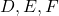be points respectively on the segments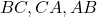such that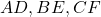concur at the point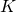. Suppose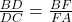and. Prove that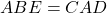.
2. Letbe a permutation (that is arrangement) of the numbers. Show that there exist two numbers. such thatand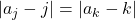.
3. A natural number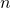is chosen strictly between two consecutive perfect square. The smaller of these two squares is obtained by subtracting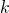fromand the larger one is obtained by addingto. Prove that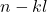is a perfect square.
4. Consider a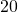-sided convex polygon K, with verticein that order. Find the number of ways in which three sides ofcan be chosen so that every pair among them has at least two sides ofbetween them. (For example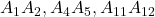is an admissible triple whileis not).
5. Let ABC be a triangle and let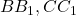be respectively the bisectors of ∠ B, ∠ C with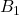on AC and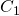on AB. Let E, F be the feet of perpendiculars drawn from A ontorespectively. Suppose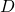is the point at which the in circle oftouches. Prove that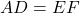.
6. Find all pairs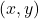of real numbers such that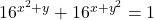.

RMO 2002 Problem 2 – Fermat’s Last Theorem as a guessing tool– Video

1. Letbe a triangle. Letbe points respectively on the segmentssuch thatconcur at the point. Supposeand. Prove that.
2. Letbe a permutation (that is arrangement) of the numbers. Show that there exist two numbers. such thatand.
3. A natural numberis chosen strictly between two consecutive perfect square. The smaller of these two squares is obtained by subtractingfromand the larger one is obtained by addingto. Prove thatis a perfect square.
4. Consider a-sided convex polygon K, with verticein that order. Find the number of ways in which three sides ofcan be chosen so that every pair among them has at least two sides ofbetween them. (For exampleis an admissible triple whileis not).
5. Let ABC be a triangle and letbe respectively the bisectors of ∠ B, ∠ C withon AC andon AB. Let E, F be the feet of perpendiculars drawn from A ontorespectively. Supposeis the point at which the in circle oftouches. Prove that.
6. Find all pairsof real numbers such that.

RMO 2002 Problem 2 – Fermat’s Last Theorem as a guessing tool– Video

This site uses Akismet to reduce spam. Learn how your comment data is processed.

### Knowledge Partner